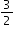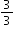# MA.3.FR.2.1

Plot, order and compare fractional numbers with the same numerator or the same denominator.

### Examples

The fractionis to the right of the fractionon a number line sois greater than.

### Clarifications

Clarification 1: Instruction includes making connections between using a ruler and plotting and ordering fractions on a number line.
Clarification 2: When comparing fractions, instruction includes an appropriately scaled number line and using reasoning about their size.

Clarification 3: Fractions include fractions greater than one, including mixed numbers, with denominators limited to 2, 3, 4, 5, 6, 8, 10 and 12.

General Information
Subject Area: Mathematics (B.E.S.T.)
Strand: Fractions
Date Adopted or Revised: 08/20
Status: State Board Approved

## Related Courses

This benchmark is part of these courses.
5012050: Grade Three Mathematics (Specifically in versions: 2014 - 2015, 2015 - 2022, 2022 and beyond (current))
7712040: Access Mathematics Grade 3 (Specifically in versions: 2014 - 2015, 2015 - 2018, 2018 - 2022, 2022 and beyond (current))
5012055: Grade 3 Accelerated Mathematics (Specifically in versions: 2019 - 2022, 2022 and beyond (current))
5012015: Foundational Skills in Mathematics 3-5 (Specifically in versions: 2019 - 2022, 2022 and beyond (current))

## Related Access Points

Alternate version of this benchmark for students with significant cognitive disabilities.
MA.3.FR.2.AP.1: Compare fractional numbers with the same denominator. Denominators are limited to 2, 3 and 4.

## Related Resources

Vetted resources educators can use to teach the concepts and skills in this benchmark.

## Formative Assessments

Comparing Fractions:

Students compare two pairs of fractions and record their comparisons using the less than or greater than symbols.

Type: Formative Assessment

The Cake Problem:

Students compare two fractional parts of two different wholes.

Type: Formative Assessment

Four-Sixths on the Number Line:

Students are asked to use a number line that includes the location of zero and one-sixth to find the location of four-sixths.

Type: Formative Assessment

One-Third on the Number Line:

Students are given four number line diagrams and asked to choose the one that correctly shows the location of one-third.

Type: Formative Assessment

Five-Eighths on the Number Line:

Students are asked to locate five-eighths on a number line that has been anchored by zero and one, but that has not yet been scaled.

Type: Formative Assessment

Three-Fourths on the Number Line:

Students are asked to scale a number line from zero to one so that they can find the location of three-fourths.

Type: Formative Assessment

## Image/Photograph

Clipart ETC Fractions:

Illustrations that can be used for teaching and demonstrating fractions. Fractional representations are modeled in wedges of circles ("pieces of pie") and parts of polygons. There are also clipart images of numerical fractions, both proper and improper, from halves to twelfths. Fraction charts and fraction strips found in this collection can be used as manipulatives and are ready to print for classroom use.

Type: Image/Photograph

## Original Student Tutorials

Fraction Camp! Fractions Greater Than 1 on a Number Line:

Joey uses his knowledge of fractions to win games at camp by knowing where fractions greater than one are located on number lines, in this interactive tutorial.

Type: Original Student Tutorial

Nature Walk: Fractions Less Than 1 on a Number Line:

Learn to use number lines to represent fractions as Emmy explores nature in this interactive tutorial.

Type: Original Student Tutorial

Finding Fractions at Camp: Fractions on a Number Line:

Joey learns about the location of unit fractions on a number line while at camp in this interactive tutorial.

Type: Original Student Tutorial

## MFAS Formative Assessments

Comparing Fractions:

Students compare two pairs of fractions and record their comparisons using the less than or greater than symbols.

Five-Eighths on the Number Line:

Students are asked to locate five-eighths on a number line that has been anchored by zero and one, but that has not yet been scaled.

Four-Sixths on the Number Line:

Students are asked to use a number line that includes the location of zero and one-sixth to find the location of four-sixths.

One-Third on the Number Line:

Students are given four number line diagrams and asked to choose the one that correctly shows the location of one-third.

The Cake Problem:

Students compare two fractional parts of two different wholes.

Three-Fourths on the Number Line:

Students are asked to scale a number line from zero to one so that they can find the location of three-fourths.

## Original Student Tutorials Mathematics - Grades K-5

Finding Fractions at Camp: Fractions on a Number Line:

Joey learns about the location of unit fractions on a number line while at camp in this interactive tutorial.

Fraction Camp! Fractions Greater Than 1 on a Number Line:

Joey uses his knowledge of fractions to win games at camp by knowing where fractions greater than one are located on number lines, in this interactive tutorial.

Nature Walk: Fractions Less Than 1 on a Number Line:

Learn to use number lines to represent fractions as Emmy explores nature in this interactive tutorial.

## Student Resources

Vetted resources students can use to learn the concepts and skills in this benchmark.

## Original Student Tutorials

Fraction Camp! Fractions Greater Than 1 on a Number Line:

Joey uses his knowledge of fractions to win games at camp by knowing where fractions greater than one are located on number lines, in this interactive tutorial.

Type: Original Student Tutorial

Nature Walk: Fractions Less Than 1 on a Number Line:

Learn to use number lines to represent fractions as Emmy explores nature in this interactive tutorial.

Type: Original Student Tutorial

Finding Fractions at Camp: Fractions on a Number Line:

Joey learns about the location of unit fractions on a number line while at camp in this interactive tutorial.

Type: Original Student Tutorial

## Parent Resources

Vetted resources caregivers can use to help students learn the concepts and skills in this benchmark.

## Image/Photograph

Clipart ETC Fractions:

Illustrations that can be used for teaching and demonstrating fractions. Fractional representations are modeled in wedges of circles ("pieces of pie") and parts of polygons. There are also clipart images of numerical fractions, both proper and improper, from halves to twelfths. Fraction charts and fraction strips found in this collection can be used as manipulatives and are ready to print for classroom use.

Type: Image/Photograph